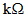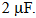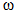# In a series resonant LCR circuit, the voltage across R is 100 volts and R =  with C =  The resonant frequency  is 200 rad/s....

In a series resonant LCR circuit, the voltage across R is 100 volts and R =with C =The resonant frequencyis 200 rad/s. At resonance the voltage across L is

Anonymous User Physics Magnetostatics 19 Apr, 2020 58 views